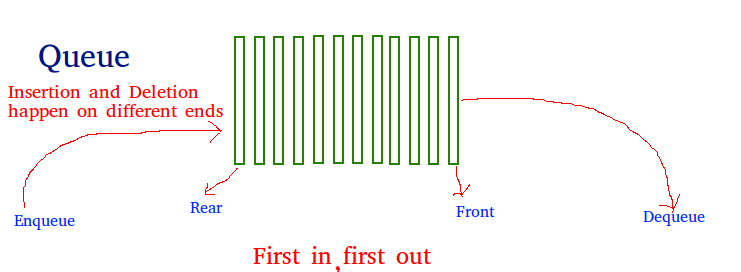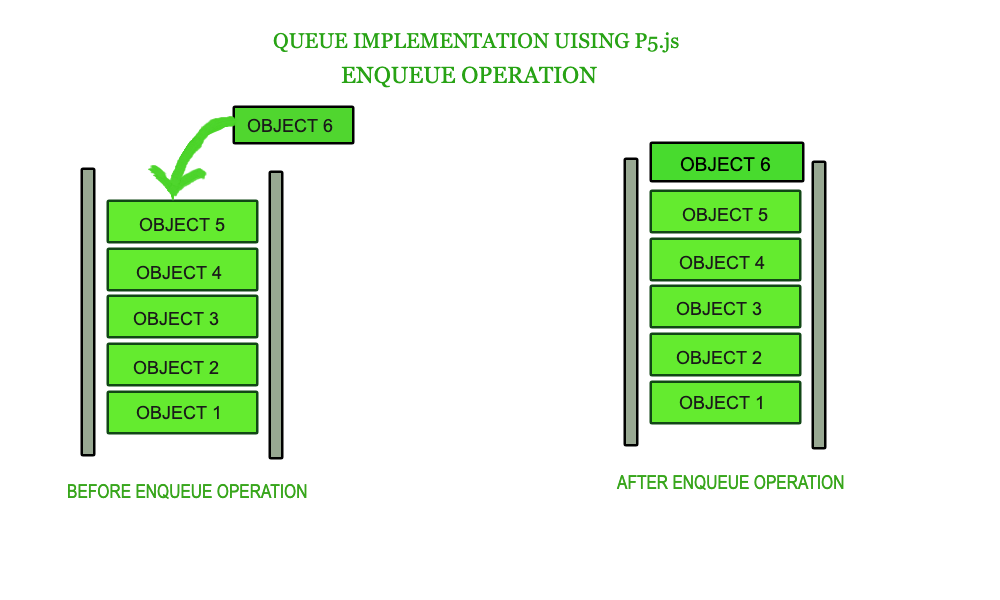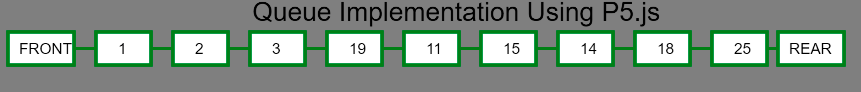# p5.js | Enqueue Operation in Queue

What is Queue?
A Queue is a linear structure which follows a particular order in which the operations are performed. The order is First In First Out (FIFO). A good example of a queue is any queue of consumers for a resource where the consumer that came first is served first. It takes constant time to add or remove an element in a queue.Queues should be used over arrays when we need to work with data in the FIFO form.

Limitation of Queue: It can access only one element at a time.In JavaScript, arrays have methods like pop and shift that defines the Queue class: Enqueue and Dequeue operations. With this, a queue can be easily implemented.

Basic skeleton of queue: Below example run using “\$node skeleton.js” command to get basic queue skelton.

 `// Define Queue function  ` `function` `Queue(array) { ` `    ``this``.array = []; ` `    ``if` `(array) ``this``.array = array; ` `} ` ` `  `// Add Get Buffer property to object  ` `// constructor which slices the array ` `Queue.prototype.getBuffer = ``function``() { ` `    ``return` `this``.array.slice(); ` `} ` ` `  `// Add isEmpty properties to object constructor ` `// which returns the length of the array ` `Queue.prototype.isEmpty = ``function``() { ` `    ``return` `this``.array.length == 0; ` `} ` ` `  `// Instance of the Queue class ` `var` `queue1 = ``new` `Queue(); ``//Queue { array: [] } ` ` `  `console.log(queue1); `

Example:

 ` ` ` ` ` `  ` ` `    ``Enqueue Operation ` `     `  `    `` ` ` `  `    `` ` ` `  `    `` ` ` ` ` `  `     ` `    `` ` ` ` ` `  `                                     `

Output:After Enqueuing ’25’ by calling queue1.enqueue(25) function of rear changes to 25.My Personal Notes arrow_drop_upCheck out this Author's contributed articles.

If you like GeeksforGeeks and would like to contribute, you can also write an article using contribute.geeksforgeeks.org or mail your article to contribute@geeksforgeeks.org. See your article appearing on the GeeksforGeeks main page and help other Geeks.

Please Improve this article if you find anything incorrect by clicking on the "Improve Article" button below.

Article Tags :

Be the First to upvote.

Please write to us at contribute@geeksforgeeks.org to report any issue with the above content.